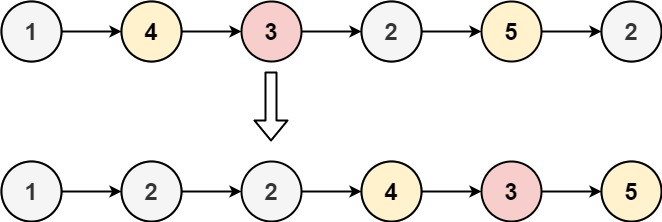86. Partition List
Medium
5.3K
618

Given the `head` of a linked list and a value `x`, partition it such that all nodes less than `x` come before nodes greater than or equal to `x`.

You should preserve the original relative order of the nodes in each of the two partitions.

Example 1:```Input: head = [1,4,3,2,5,2], x = 3
Output: [1,2,2,4,3,5]
```

Example 2:

```Input: head = [2,1], x = 2
Output: [1,2]
```

Constraints:

• The number of nodes in the list is in the range `[0, 200]`.
• `-100 <= Node.val <= 100`
• `-200 <= x <= 200`
Accepted
442.6K
Submissions
852.1K
Acceptance Rate
51.9%

Seen this question in a real interview before?
1/4
Yes
No

Discussion (0)

Related Topics